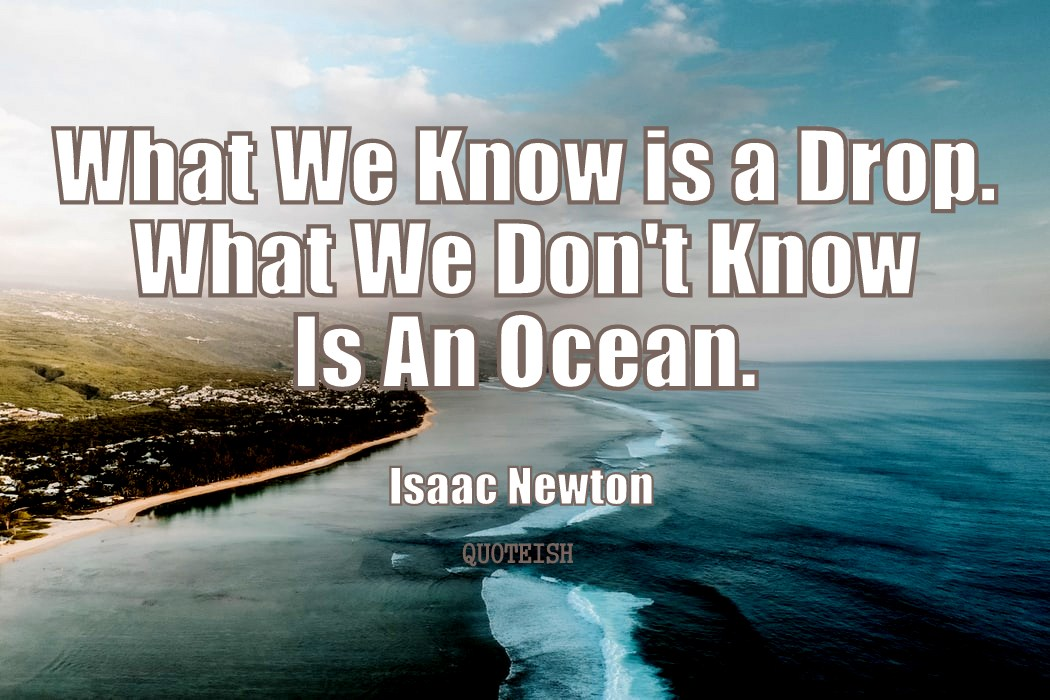All Quotes, Isaac Newton

# 19 Isaac Newton quotes

Sir Isaac Newton was an English mathematician, physicist, and author. Newton is widely recognised as one of the most influential scientists of all time. He is regarded as a key figure in the scientific revolution. Newton formulated the laws of motion and universal gravitation. Newton built the first practical reflecting telescope. This is a Quoteish quote tribute to the scientist Isaac Newton with 19 of his most influential quotes on art, patience and explanation.What we know is a drop. What we don't know is an ocean. - Isaac Newton
We build too many walls and not enough bridges. - Isaac Newton
Errors are not in the art but in the artificers. - Isaac Newton
The centre of the system of the world is immovable. - Isaac NewtonGenius is patience. - Isaac Newton
Nature is pleased with simplicity. And nature is no dummy. - Isaac Newton
I can calculate the motion of heavenly bodies, but not the madness of people. - Isaac Newton
Tact is the art of making a point without making an enemy. - Isaac NewtonWhat goes up must come down. - Isaac Newton
Truth is ever to be found in simplicity, and not in the multiplicity and confusion of things. - Isaac Newton
It is the weight, not numbers of experiments that is to be regarded. - Isaac Newton
Tact is the knack of making a point without making an enemy. - Isaac Newton
No great discovery was ever made without a bold guess. - Isaac Newton
Live your life as an Exclamation rather than an Explanation. - Isaac Newton
To explain all nature is too difficult a task for any one man or even for any one age. - Isaac Newton
For every action, there is an equal and opposite reaction. - Isaac Newton
If others would think as hard as I did, then they would get similar results. - Isaac Newton
Truth is the offspring of silence and meditation. - Isaac Newton
Nature is exceedingly simple and harmonious with itself. - Isaac Newton